CATEGORIES:

# Since, the point belongs to the plane, its coordinates satisfy the general equation of the plane, andz M3

M2 y

M1

х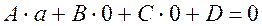.

Therefore,;.

By analogy, we obtain;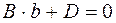;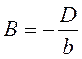,;;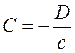.

Substituting the obtained values of the coefficients А,B, and C into the general equation of the plane, we obtain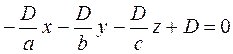,

which gives, after the reduction by D, the three-intercept equation of the plane: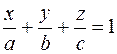.

The equation of a plane passing through three points. Suppose given, three points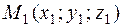,, and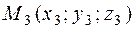. As is known form elementary geometry, there exists a unique plane passing through these points. It is required to write its equation.

Following the general scheme, of we take an arbitrary point M(x;y;z) in the plane.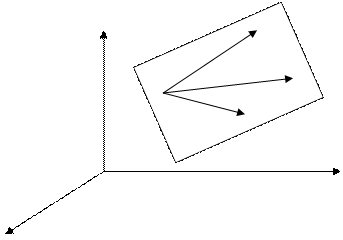z M2

M1 M(x;y;z)

M3

y

0

x

The characteristic feature of a plane is that if a point М belongs to the plane, then the three vectors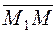={x–x1;y–y1; z–z1},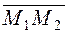= {x2–x1;y2–y1;z2–z1},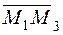={x3–x1;y3–y1;z3–z1},

are coplanar.

Therefore, the triple product of these vectors must be zero: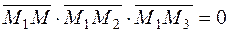.

Expressing the triple product in terns of coordinates, we obtain an equation of the plane, passing through the three given points:. (24)

Example. Write an equation of the plane passing through the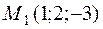;;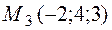.

By formula (24), we have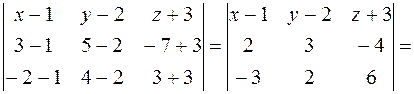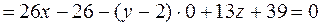or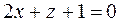.

The Angle between Planes

Consider the planes given by the equations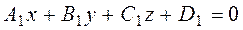;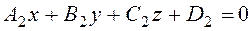,

which have normal vectorsand. Using inner product, we find the cosine of the angle:.

The condition for two planes to be parallel coincides with the condition for the normal vectors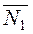and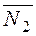to be collinear: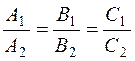.

The perpendicularity conditioin coincides with the perpendicularity condition for the vectorsand:

(·)=0, i.e., A1A2+B1B2+C1C2=0.

Example 1. Show that the following planes are parallel or perpendicular: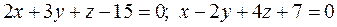.

The planes are perpendicular, because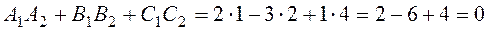.

Example 2. Write an equation of the plane passing through the point М0(–1;2; 4) and parallel to the plane 6x-7y+5z+11=0.

The normal vector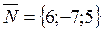is normal to the required plane also. We have

A(x–x0)+B(y–y0)+C(z–z0)=0,

6(x+1)–7(y–2)+5(z–4)=0; 6x–7y+5z=0.The normal equation of a plane. Consider a plane. Let us draw the perpendicular ОР from the origin to this plane. Let a,b, and g be the angels between this perpendicular and the coordinate axes x,y, and z, and let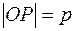. It is required to write an equation of this plane.

z

P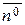M(x,y,z)

0 y

x

Take an arbitrary point M(x;y;z) in the plane and consider the radius-vector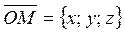. The unit vector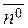on the perpendicular ОР has the coordinates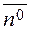={cos α; cos b; cos g}.

For any point М in the plane, the projection of the vectoron the unit vectorequals р: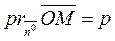.

Consider the scalar product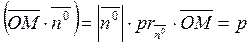,

or, in coordinate form,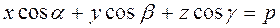.

Thus, we have obtained the normal equation of the plane: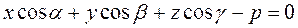. (25)

The normalizing factor. Consider the plane given by the general equation Ax+By+Cz+D=0.

It is required to reduce this equation to the normal form (25).

Definition. Number m is called the normalizing factor if the equation multiplied by it is normal.

To find the normalizing factor, we multiply the general equation of the plane by a number m term by term:

mAx+mBy+mCz+mD=0.

This equation is normal if the two normality conditions hold, i.e.,

1. (mA)2+(mB)2+(mC)2=1,

2. mD<0.

From the first condition, taking out m2 and extracting the square root, we find the normalizing factor.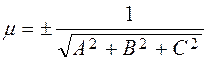.

The sign opposite to that of the free term D must be taken.

Date: 2015-01-02; view: 774

 <== previous page | next page ==> Analytic geometry in space. Vectors. Simple operations with vectors. The scalar, vector and mixed product of vectors | STRAIGHT LINES IN SPACE
doclecture.net - lectures - 2014-2020 year. Copyright infringement or personal data (0.004 sec.)